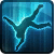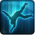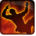# Force SlamConditions

Used by

## Related effects, buffs and debuffs

Please click on an effect below to view its details.

• [0s] [not displayed]Effect #1

 Slot: Buff Duration: 0s Tick rate: does not tick # occurrences: 0
• On Apply
• [target override]

Perform the following actions:

• Add effect #2 to TARGET from TARGET
• [4s]Knockdown
Prone and unable to act.

 Slot: Debuff Duration: 4s Tick rate: 1s # occurrences: 0 Tags: 8255977782224933496, tag.​abl.​debuff.​stun, tag.​abl.​npc.​immobilized Conditions: Can only by called by other effects
• On Apply

Perform the following actions:

• Play appearance epp . jedi_consular . force_slam . force_slam, dependent on calling effect
• Stun the TARGET
• On Apply

Only when the following conditions are met:

• If difficulty is set to 16-man

Perform the following actions:

• Spell Damage
- Unknown (609) = (bool) false
- Damage Type = (int) 4
- Slot = (int) 1
- Spell Type = (int) 3
- Level Cap = (int) 0
- Amount Max = (float) 0
- Amount Min = (float) 0
- Amount Percent = (float) 0
- Threat Percent = (float) 0
- Standard Health Percent Max = (float) 0.02
- Standard Health Percent Min = (float) 0.02
- Amount Modifier Percent = (float) 0
- Coefficient = (float) 1
- Health Steal Percentage = (float) 0
• When effect ticks the 1th time
• [target override]

Only when the following conditions are met:

Perform the following actions:

• Unknown (164)
- Ability Spec = (int) 16140942419358413020
- From Actor = (int) 3
- To Actor = (int) 3
• On Apply

Only when the following conditions are met:

• If difficulty is set to 16-man

Perform the following actions:

• Spell Damage
- Unknown (609) = (bool) false
- Damage Type = (int) 4
- Slot = (int) 1
- Spell Type = (int) 3
- Level Cap = (int) 0
- Amount Max = (float) 0
- Amount Min = (float) 0
- Amount Percent = (float) 0
- Threat Percent = (float) 0
- Standard Health Percent Max = (float) 0.6
- Standard Health Percent Min = (float) 0.06
- Amount Modifier Percent = (float) 0
- Coefficient = (float) 1
- Health Steal Percentage = (float) 0
• On Apply

Only when the following conditions are met:

• If difficulty is set to 8-man SM

Perform the following actions:

• Spell Damage
- Unknown (609) = (bool) false
- Damage Type = (int) 4
- Slot = (int) 1
- Spell Type = (int) 3
- Level Cap = (int) 0
- Amount Max = (float) 0
- Amount Min = (float) 0
- Amount Percent = (float) 0
- Threat Percent = (float) 0
- Standard Health Percent Max = (float) 0.04
- Standard Health Percent Min = (float) 0.04
- Amount Modifier Percent = (float) 0
- Coefficient = (float) 1
- Health Steal Percentage = (float) 0
• On Apply

Only when the following conditions are met:

• If difficulty is set to 8-man HM

Perform the following actions:

• Spell Damage
- Unknown (609) = (bool) false
- Damage Type = (int) 4
- Slot = (int) 1
- Spell Type = (int) 3
- Level Cap = (int) 0
- Amount Max = (float) 0
- Amount Min = (float) 0
- Amount Percent = (float) 0
- Threat Percent = (float) 0
- Standard Health Percent Max = (float) 0.04
- Standard Health Percent Min = (float) 0.04
- Amount Modifier Percent = (float) 0
- Coefficient = (float) 1
- Health Steal Percentage = (float) 0